Courses

# Special Techniques (Part - 1) Electrical Engineering (EE) Notes | EduRev

## Electrical Engineering (EE) : Special Techniques (Part - 1) Electrical Engineering (EE) Notes | EduRev

The document Special Techniques (Part - 1) Electrical Engineering (EE) Notes | EduRev is a part of the Electrical Engineering (EE) Course Electromagnetic Theory.
All you need of Electrical Engineering (EE) at this link: Electrical Engineering (EE)

Method of Images

The uniqueness theorem for Poission’s or Laplace’s equations, which we studied in the last couple of lectures, has some interesting consequences. Frequently, it is not easy to obtain an analytic solution to either of these equations. Even when it is possible to do so, it may require rigorous mathematical tools. Occasionally, however, one can guess a solution to a problem, by some intuitive method. When this becomes feasible, the uniqueness theorem tells us that the solution must be the one we are looking for.

One such intuitive method is the “method of images” a terminology borrowed from optics. In this lecture, we illustrate this method by some examples.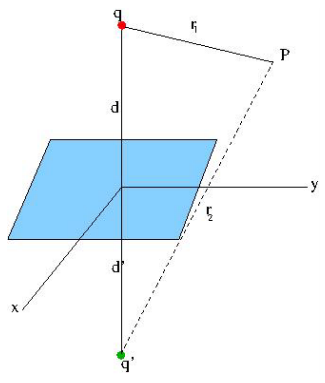Consider an infinite, grounded conducting plane occupying which occupies the x-y plane. A charge q is located at a distance d from this plane, the location of the charge is taken along the z axis. We are required to obtain an expression for the potential everywhere in the region z > 0 , excepting of course, at the location of the charge itself. Let us look at the potential at the point P which is at a distance r1 from the charge q (indicated by a red circle in the figure).

Instead of solving the problem from the first principles, let us suppose there existed an “image charge” q’ at a distance d’  “below” the plane. This is very similar to the image of an object located in front of a mirror, the image of the object is “behind” the mirror. The image is virtual because we cannot capture the image on a screen behind the mirror. In the same way, the image charge is located at a virtual distance and the charge itself is fictitious. Let the point P be at a distance r2 from the image charge. We take the origin on the plane, as shown. Let the position of P be (x,y,z).

The potential φ1(P) at P due to the real charge q and φ2(P) due to the image charge q’ is given by superposition,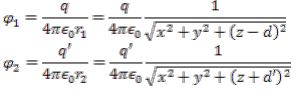The potential P is given by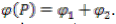Note that this expression for the potential satisfies the Laplace’s equation at a general point P above the conducting plane, since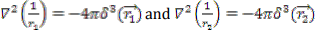and at a point P, neither of the distances is zero so that the delta function vanishes. Let us impose the boundary condition of the potential being zero on the boundary, We must have, on the plane,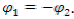Since the distances are positive, the chargesq and  q’ must have opposite sign. V Further, we must have, substituting z = 0 for the position of the conducting plane,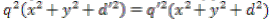Rewriting this as,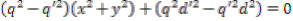This equation must be satisfied at all points on the conducting plane, I.e for arbitrary values of x,y. Thus the two terms of the above equation must be separately zero. This gives us the solution,Thus the image charge is equal and opposite to the object charge and, like in the case of optical images, the image distance is equal to the object distance.

It is important to realize that the field exists only in the region above the conducting plane. The expression for potential which satisfies Laplace’s equation everywhere above the plane and also satisfies the boundary condition on the plane, is given by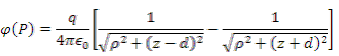where we have, because of obvious reasons, switched to a cylindrical coordinates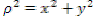as the square of the distance of the foot of the perpendicular from the point P onto the plane from the origin.

The electric field is obtained as the negative gradient of the potential, and is given by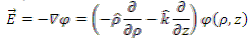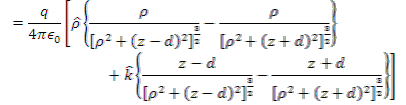We emphasize that this expression is valid only in the region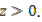From this expression for the electric field, we can obtain the charge density induced on the surface. Remembering that charge density is given by the normal component of the electric field, we only need to evaluate the z component of the electric field at z=0,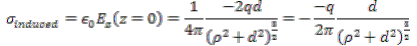The total induced charge is obtained by integrating this expression in the entire xy plane,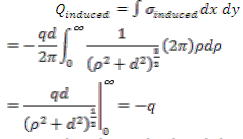Thus the total induced charge has the same magnitude as the real charge, though of opposite sign. In the following figure we have plotted the charge density as a function of x-y coordinates on the plane for some representative distances of the object charge. One can see that the magnitude of the charge density is maximum at a point on the plane directly opposite to the real charge and decreases as the distance from this point increases.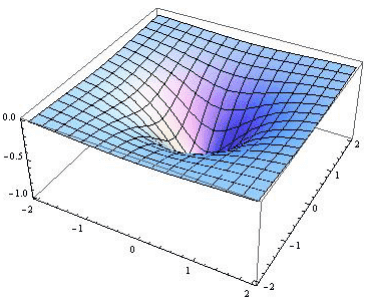The following figure shows the lines of force and equipotentials. As expected the lines of force strike the conducting plane normally. The equipotentials are spheres (shown as circles)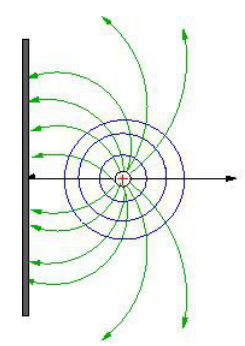Thus in the above problem we have replaced the original problem of a charge and a conducting plane by the charge and an image charge and eliminated the conducting plane altogether. The solution obtained satisfies the boundary condition that at z=0, the potential is zero. The solution does not make sense in the region z < 0 but that is immaterial because we did not seek the solution in that region in any case! The electric field on the plane z = 0is purely along the vertical plane because, the object and the image charges being equidistant from a point on the conducting plane, the horizontal components cancel by symmetry. The uniqueness theorem guarantees that this is the only possible solution.

Let us calculate the field that is generated at the position of the real charge due to the induced charges. For this we take the general expression for the electric field and consider only the contribution due to the image charge, given by the second term within each curly bracket. As the real charge is located at p = 0, z = d, only the normal component gives non-zero contribution,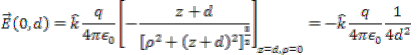Thus the force on the charge due to the charges induced on the surface of the conductor is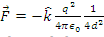which is the same as the force exerted by the image charge on the real charge (located at a distance 2d from each other.

It is interesting to calculate field at the surface due to the charge q. In this case, we do a bit of hand waving and consider only half of the field that we have calculated. This is because the image charge being fictitious does not create a field on the surface of the conductor. The force on the conductor is then given by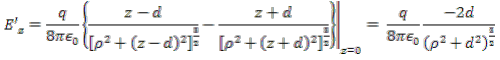The force exerted on the surface is then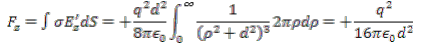which, as expected from Newton’s third law, is equal and opposite to the force exerted on the charge by the surface.

We can now proceed to calculate the electrostatic energy of the system. This is just the work done in bringing the charge from infinity to its position on the z axis. We already have an expression for the force exerted on the charge when it is at a distance d from the surface. It is a simple matter to get the expression for the force when the charge is at a distance z along the z axis. Since the electrostatic force is conservative, we bring the charge along the z axis. The work done is then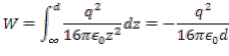Example 1

Consider two semi infinite grounded, conducting planes intersecting along the z axis, which is taken out of the plane of the paper (which is the xy plane). A charge q is located at a point (a, b) Calculate the force on the charge q due to the charges induced on the surfaces of the planes.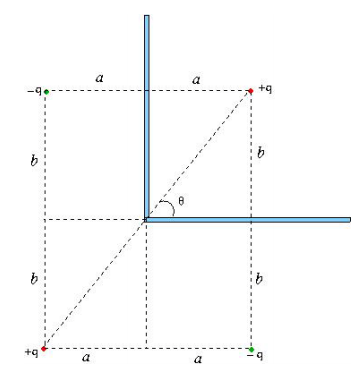The image charges are also line charges of charge density -λ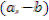and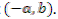In addition, there is a line charge of density + λ at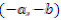The potential due to a line charge parallel to z axis is independent of z coordinate and only depends on the distance p of the point from the line charge, being given by,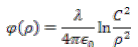where C is a constant and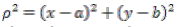where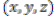is the coordinate of the point P where the potential is sought. Similar expressions for the potential due to the image charges can be written down and the total potential obtained by superposition. Thus, the net potential atis given by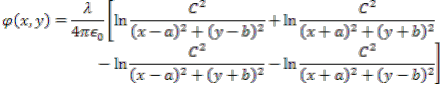It can be seen that the solution satisfies the boundary condition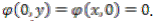Note that the tangential components on the y = 0 plane is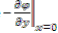and that on the x = 0 plane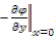are zero, as expected. The normal components, from which we can infer the charge densities are along the positive y direction for the former and along the positive x direction for the latter.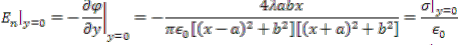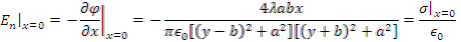In the following figure we have plotted the charge densities for different locations of the given charged line indicated on the graph. Consider the situation where the line charge is at the point (a = 2, b= 1). The axes are the coordinates of points on the plane.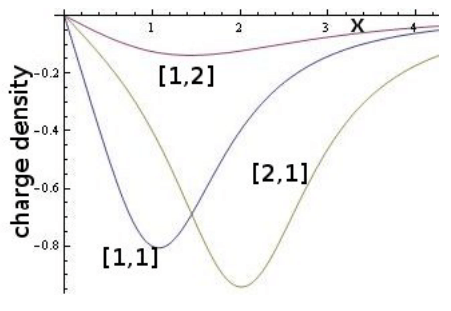Note that the charge density is maximum on the plane at the point (2,1) and spreads out when one goes away from this point. If you reduce the x distance to, say, [1,1], the charge density has a smaller peak and spreads out more.

The total linear charge density on the y=0 plane can be calculated by integrating the charge density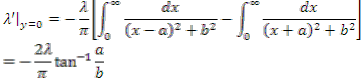The linear charge density on the x=0 plane can be written down by symmetry to be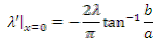Adding these and using the identity tan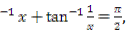we get the total linear charge density on the two planes to be -λ.

The method of images is not restricted to only planar geometry. We will take up different application in the next lecture. (Part of the video for application of method of images applied to spherical conductors appears in this unit but the text for the entire problem appears along with Lecture 18].

Tutorial Assignment

1. A point charge is located at a distance d above a grounded, infinite, conducting plane. Let P be the foot of the perpendicular from the position of the charge on to the plane. Find the radius of a circle centred at P in which one quarter of all the induced charges remain.

2. A point charge q is located at a point P along the bisector of the angle between two semi-infinite grounded conducting planes at a distance d from the intersection of the planes. The angle between the planes is 600. Determine all the image charges. Find the potential at a point S, located at a distance r from the intersection along the line joining P and S.

Solutions to Tutorial assignment

1. We had shown that the charge density is given by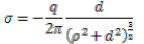So, the charge induced in a radius R is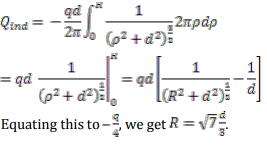2. The image charges along with the charge q forms a regular hexagon of side d, as shown. The image of q at P is –q at P1, the image of –q at P1 is +q at P2, the image of +q at P2 is –q at P3, the image of –q at P3 is +q at P4 and the image of +q at P4 is –q at P5, as shown in the figure.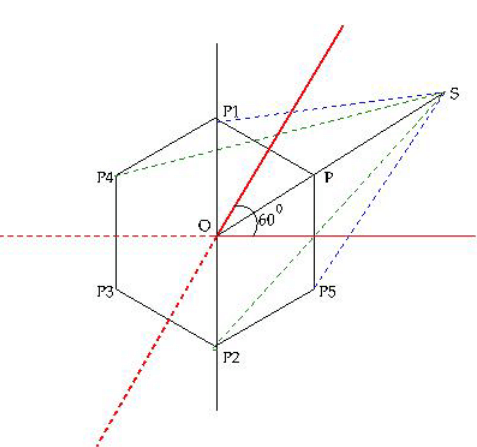Note that the distance of P1 and P5 from S is given by triangle law is ( since OP1=OP5=d)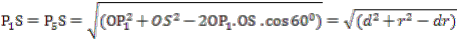Similarly,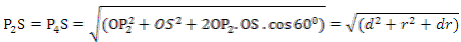The distances PS = r - d and P3S = R + d.

Using these distances the potential at S can be written as (the signs of the terms are according whether a charge +q or –q appears)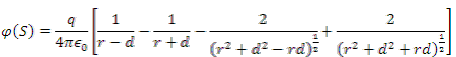Expand each term in powers of 1/r, keeping terms up to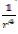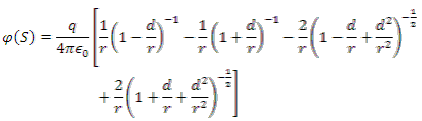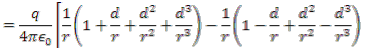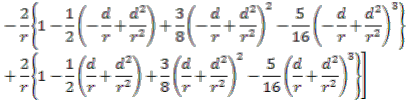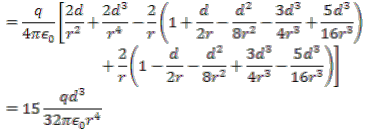Self Assessment Quiz

1. A charge q is located z = d at and a second charge -2q at z = 2d above an infinite grounded conducting plane occupying the xy plane at z = 0. Calculate the force exerted on the charge q.

2. A point charge q is located at a distance d above a grounded, infinite, conducting plane. A second charge -q is free to move along the perpendicular from the position of the charge q on to the plane. Where should this charge –q be placed so that the net force on it is zero?

Solution to Self Assessment Quiz

1. The charge q and the charge -2q gives rise to two images, the former an image charge located at a distance d below the plane and the latter an image +2q at a distance  2d below the plane. Thus the force (away from the plane) on the charge q is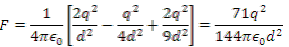2. The image charges due to the charges q and -q are shown. Let the charge -q be at a distance x from the plane.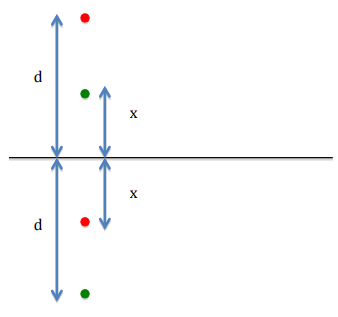Force on –q in upward direction is to be equated to zero. This gives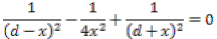This simplifies to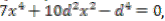which has the solution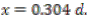Offer running on EduRev: Apply code STAYHOME200 to get INR 200 off on our premium plan EduRev Infinity!

,

,

,

,

,

,

,

,

,

,

,

,

,

,

,

,

,

,

,

,

,

;